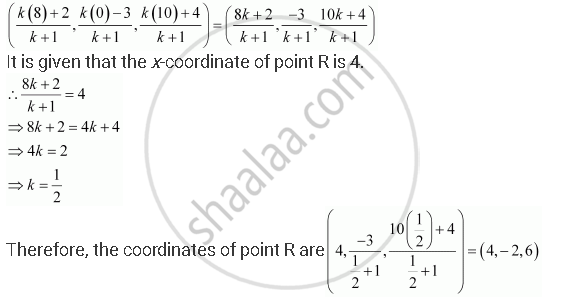# A Point R With X-coordinate 4 Lies on the Line Segment Joining the Pointsp (2, –3, 4) and Q (8, 0, 10). Find the Coordinates of the Point R. - Mathematics

A point R with x-coordinate 4 lies on the line segment joining the pointsP (2, –3, 4) and Q (8, 0, 10). Find the coordinates of the point R.

[Hint suppose R divides PQ in the ratio k: 1. The coordinates of the point R are given by ((8k + 2)/(k+1), (-3)/(k+1), (10k + 4)/(k+1))

#### Solution

The coordinates of points P and Q are given as P (2, –3, 4) and Q (8, 0, 10).

Let R divide line segment PQ in the ratio k:1.

Hence, by section formula, the coordinates of point R are given byConcept: Three - Dimensional Geometry - Coordinates of a Point in Space
Is there an error in this question or solution?

#### APPEARS IN

NCERT Class 11 Mathematics
Chapter 12 Introduction to Three Dimensional Geometry
Q 5 | Page 279